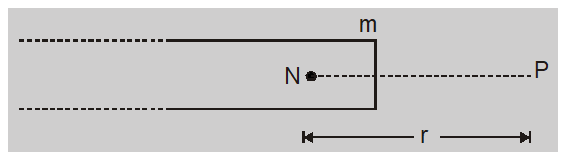Most Affordable JEE | NEET | 8,9,10 Preparation by Kota's Top IITian Doctor Faculties

# Coulomb's law of Magnetism || Magnetism and Matter Class 12, JEE & NEET`
Magnetism and Matter Class 12 Notes will help you in your Exam Preparation and will also help in scoring good! The property of any object by virtue of which it can attract a piece of iron or steel is called Magnetism. Here we will study about the Coulomb's Law in Magnetism, Magnetic Flux Density, and Pole Strength.

## Coulomb's Law in MagnetismIf two magnetic poles of strengths ${{m_1}}$ and ${{m_2}}$ are kept at a distance r apart then the force of attraction or repulsion between the two poles is directly proportional to the product of their pole strengths and inversely proportional to the square of the distance between them

$$F \propto {{{m_1}{m_2}} \over {{r^2}}}\quad or\,\,F = {{{\mu _0}} \over {4\pi }}{{{m_1}{m_2}} \over {{r^2}}}$$

Where $\frac{\mu_{0}}{4 \pi}=10^{-7} Wb A ^{-1} m ^{-1}=10^{-7}$ henry/m where $\mu_{0}$ is permeability of free space.

## Magnetic Flux DensityThe force experienced by a unit north pole when placed in a magnetic field is called magnetic flux density or field intensity at that point

$\overrightarrow{ B }=\frac{\overrightarrow{ F }}{ m }=\frac{\mu_{0}}{4 \pi} \frac{ m }{ r ^{2}} \hat{ r }$

This is the magnetic field produced by a pole of strength m at distance r.

## Pole Strength

In relation $F=\frac{\mu_{0}}{4 \pi} \frac{m_{1} m_{2}}{r^{2}}$

If $m _{1}= m _{2}= m , r =1 m$ and $F =10^{-7} N$

Then $10^{-7}=10^{-7} \times \frac{ m \times m }{1^{2}}$ or $m^{2}=1$ or $m=\pm 1$ ampere metre (A-m)

The strength of a magnetic pole is said to be one ampere meter if it repels an equal and similar pole with a force of $10^{-7}$ N when placed in vacuum (or air) at a distance of one meter from it.

The pole strength of the north pole is defined as the force experienced by the pole when kept in a unit magnetic field.

$m =\frac{\overrightarrow{ F }}{\overrightarrow{ B }}$
1. Pole strength is a scalar quantity with dimension $M^{0} L^{1} T^{0} A^{1}$
2. The unit is newton/Tesla or ampere meter.
3. The pole strength depends on the nature of the material of the magnet, the state of magnetization (with an upper limit called saturation), and the area of cross-section.
4. The north pole experiences a force in the direction of the magnetic field while the south pole experiences force opposite to the field.

About eSaral At eSaral we are offering a complete platform for IIT-JEE & NEET preparation. The main mission behind eSaral is to provide education to each and every student in India by eliminating the Geographic and Economic factors, as a nation’s progress and development depends on the availability of quality education to each and every one. With the blend of education & technology, eSaral team made the learning personalized & adaptive for everyone.